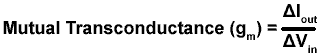# Conductance

• After studying this section, you should be able to:
• Describe the property of Conductance (G)
• Describe the property of Mutual transconductance (gm)

## The Opposite of Resistance

The Ohms Law formula for resistance is R = V/I. If this for R formula is inverted it would become R = I/V. This is still a useful formula, but NOT for resistance. Resistance is a property that, as it increases, reduces current flow. I/V therefore must give a unit that, as it increases also INCREASES current flow, exactly the opposite effect to resistance. This unit must be proportional to current. (Resistance is INVERSELY proportional to current).

## Conductance

This property given by I/V is called CONDUCTANCE because the larger its value, the more a circuit conducts (passes more current). The property of Conductance is given the letter G and is measured in units of Siemens (S). As conductance is the opposite of resistance it can also be calculated as the RECIPROCAL of resistance.Enter the resistance of a circuit (in Ohms) into a scientific calculator and simply press the reciprocal button (labelled 1/x or sometimes x −1) and you have Conductance in Siemens, note that the symbol for Siemens a capital S (small s is used for seconds). Conductance is not widely used in electronics calculations, resistance being generally a more useful property.

## Transconductance

Conductance is used however in connection with Field Effect Transistors (FETs) used as amplifiers and with operational amplifier integrated circuits (Op Amp ICs). In these devices a change in output current is related to a change in input voltage by a ratio called the Transconductance or mutual Transconductance of the (amplifier) device.

Mutual Transconductance is given the symbol gm and gives an indication of the gain of a device (i.e. how much it amplifies a signal). The formula for gm is given below and relates a change (Δ) in output Current (Iout) to a change of input Voltage (Vin).Top of Page.>